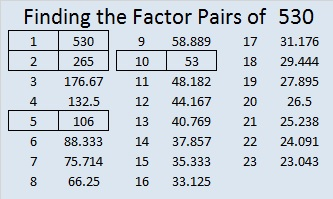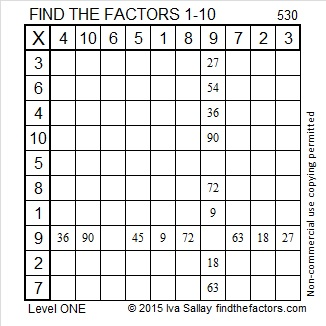# 530 and Level 1

The first three perfect numbers are 6, 28, and 496. Their sum is 530.

Can you find the greatest common factors for each of these Pythagorean triples?

• 46-528-530
• 192-494-530
• 280-450-530
• 318-424-530Print the puzzles or type the solution on this excel file: 10 Factors 2015-06-22

—————————————————————————————————

• 530 is a composite number.
• Prime factorization: 530 = 2 x 5 x 53
• The exponents in the prime factorization are 1, 1, and 1. Adding one to each and multiplying we get (1 + 1)(1 + 1)(1 + 1) = 2 x 2 x 2 = 8. Therefore 530 has exactly 8 factors.
• Factors of 530: 1, 2, 5, 10, 53, 106, 265, 530
• Factor pairs: 530 = 1 x 530, 2 x 265, 5 x 106, or 10 x 53
• 530 has no square factors that allow its square root to be simplified. √530 ≈ 23.02172886644—————————————————————————————————